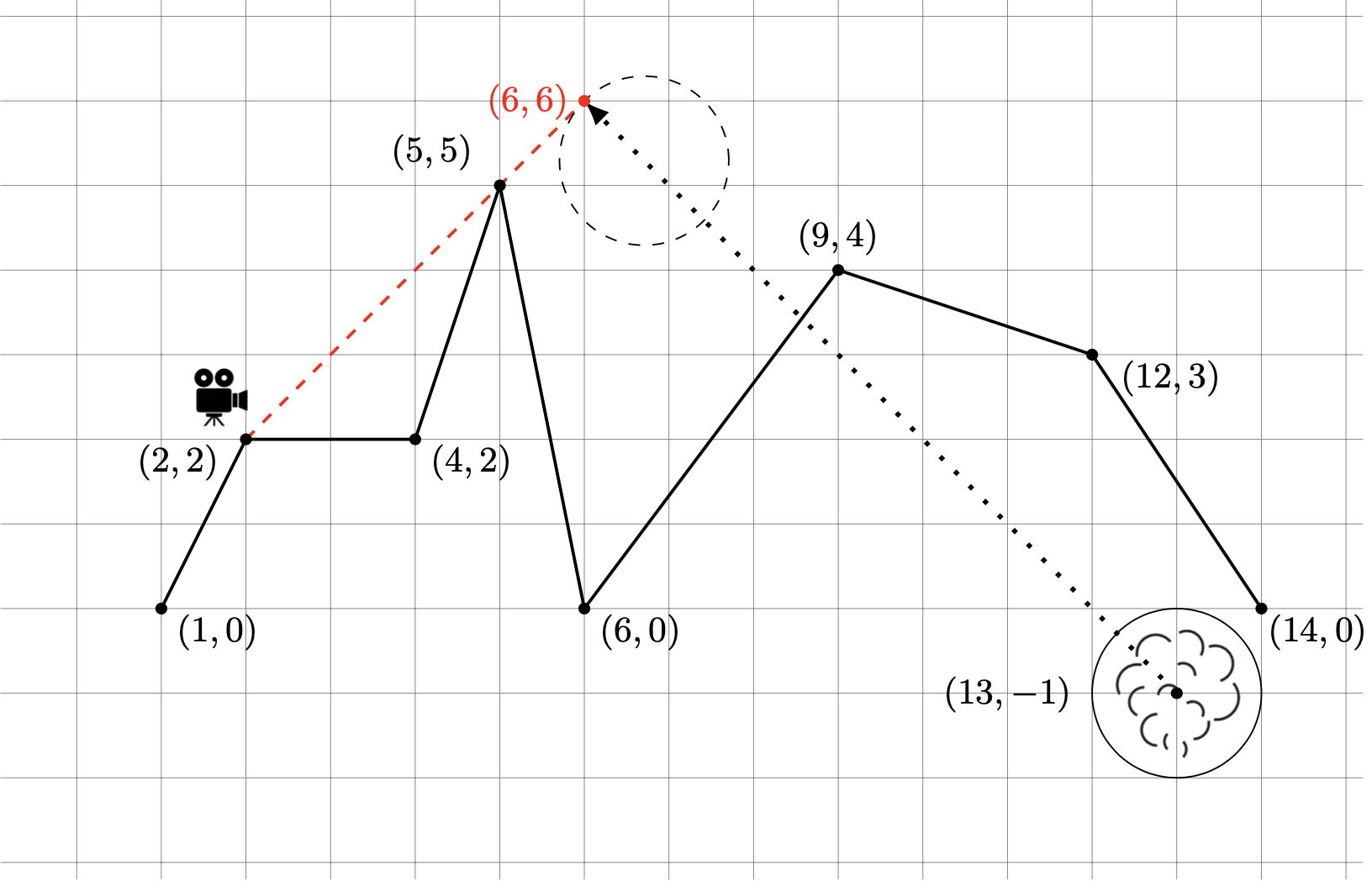시간 제한메모리 제한제출정답맞힌 사람정답 비율
1 초 1024 MB0000.000%

## 문제

Meteorologist Wendy Wynne Blose and geologist Maddy Morfik are in the Porous Mountains of Central Asia, studying mild eruptions of superheated smoke and steam from below ground. Unlike more dramatic eruptions such as the one that blew the top off Mount St. Helens in $1980$, these milder events involve the expelling of steam through many tiny fissures in the (extremely porous) ground, preserving the ground surface while producing a visible cloud that rises through the air. Wendy and Maddy would like to capture the path of such a cloud on video.

Like all good scientists, they have made some simplifying assumptions. They assume the cloud will be spherical, of known radius, and will travel at a fixed known speed along a straight line. They also assume that the cloud will form underground and will be expelled in a certain direction, always able to pass through the porous mountain rock with no change in speed, direction, or shape. The scientists have delicate instruments that are quite good at predicting when such an underground steam cloud will be expelled. Finally, they have a model of the surrounding mountain range in the form of a continuous piecewise-linear approximation.

For safety reasons they will set up a video camera and a timer that will turn on the camera at the instant they predict the steam cloud will first become visible from the camera's vantage point; then they will go to a safe location far away and wait until the recording is complete. Figure H.1 shows a typical scenario. The camera at $(2,2)$ needs to be aimed in the direction of the peak at $(5,5)$ in order to pick up the cloud at the moment it first becomes visible at $(6,6)$. If the cloud has a radius of $1$ unit and is travelling in the direction of the vector $[-1,1]$ (i.e., at an angle of $135$ degrees) from its underground starting point at $(13,-1)$ at a speed of one unit per second, the camera should be turned on after $8.899$ seconds.Figure H.1: First sample input

In order to get maximum recording time from the camera's battery, they need to know the precise time to turn on the camera. That's where you come in.

## 입력

The first line contains an integer $n$ $(2 \leq n \leq 1\,000$, the number of line segments defining the mountain range, followed by $n+1$ integer pairs $(x_i,y_i)$ ($-5\,000 \leq x_0 < \cdots < x_n \leq 5\,000$, $0 \leq y_i \leq 5\,000$), where $(x_{i-1},y_{i-1})$ and $(x_i,y_i)$ define the endpoints of the $i$-th line segment. No three consecutive points will be collinear. The second line contains seven integers $c$, $sx$, $sy$, $r$, $dx$, $dy$, and $v$, where $c$ ($x_0 \leq c \leq x_n$) specifies the $x$-coordinate of the camera's location along the mountain range, $(sx,sy)$ ($x_0 \leq sx \leq x_n$, $-5\,000 \leq sy \leq 5\,000$) specifies the initial underground location of the steam cloud, $r$ ($1 \leq r \leq 5\,000$) is the radius of the cloud, $dx$ and $dy$ ($-5\,000 \leq dx \leq 5\,000$, $1 \leq dy \leq 5\,000$) specify the direction of travel of the steam in vector form ($dy$ units of vertical distance for each $dx$ units of horizontal distance), and $v$ ($1 \leq v \leq 1\,000$) is the velocity of the cloud in units per second.

## 출력

Output a single real number $t$, the time, in seconds, when the camera should be turned on, i.e., when the first point of a non-zero volume of visible cloud can be seen at a location between $x_0$ and $x_n$. Assume the cloud begins moving from its starting point at time $0$ and maintains a constant speed and direction from that point on. The cloud will first emerge from underground at a point whose $x$-coordinate is between $x_0$ and $x_n$. If it will never be possible for the camera to view the cloud at a point whose $x$-coordinate lies between $x_0$ and $x_n$, output the value $-1$. Answers should be accurate with an absolute or relative error of $10^{-3}$.

## 예제 입력 1

7 1 0 2 2 4 2 5 5 6 0 9 4 12 3 14 0
2 13 -1 1 -1 1 1


## 예제 출력 1

8.899